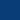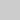## Efficient techniques for tree similarity join

### (master thesis or bachelor project)

Many applications deal with hierarchical data. Examples are XML and JSON files which can be represented as trees. In data integration, a join matches elements that are common to two data sources. Since elements are represented slightly different in each source, an approximate join must be used to do the matching. Such a join searches for pairs of similar items. When dealing with massive datasets or large tree instances, efficient techniques must be developed to compute the join in reasonable time.

In a similarity join, a threshold value is provided which says how much two trees must be similar to be part of the result. Computing the similarity of trees is very complex and requires expensive algorithms. Instead of calculating the similarity value for each pair of trees in the datasets, a filtering step is usually applied. Then, pairs of trees are tested against a filter, which is computationally cheap. The filter disregards the tree pairs which certainly exceed the threshold. At the end, the pairs which pass the filter (usually much less than without the filter) are verified by computing the similarity value.

An example of tree similarity measure is the tree edit distance which says how to transform one tree into another by deleting, inserting, and relabelling nodes. Since, the tree edit distance has cubic runtime, efficient and effective filtering techniques must be developed.

The goal of this thesis is to develop efficient methods for tree similarity joins based on the tree edit distance with an emphasis on both filter and verification step. The work consists of the following steps:

• study tree similarity joins literature,
• develop efficient and effective filtering techniques based on lower and upper bounds which approximate the tree edit distance value,
• design efficient verification methods for similar tree pairs,
• implement and experimentally evaluate the resulting algorithms.

Contact: Mateusz Pawlik, Nikolaus Augsten

AlgorithmikProgrammierungBenchmarkingInnovation